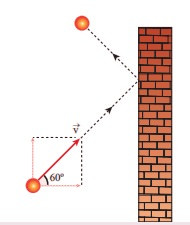# UNIT IX: Kinetic Theory of Gases - Online Test

Q1.  A particle of mass m is moving with speed u in a direction which makes 60° with respect to x axis. It undergoes elastic collision with the wall. What is the change in momentum in x and y direction?Explaination / Solution:
No Explaination.

Q2. A sample of ideal gas is at equilibrium. Which of the following quantity is zero?
Explaination / Solution:
No Explaination.

Q3. An ideal gas is maintained at constant pressure. If the temperature of an ideal gas increases from 100K to 1000K then the rms speed of the gas molecules
Explaination / Solution:
No Explaination.

Q4. Two identically sized rooms A and B are connected by an open door. If the room A is air conditioned such that its temperature is 4° lesser than room B, which room has more air in it?
Explaination / Solution:
No Explaination.

Q5. The average translational kinetic energy of gas molecules depends on
Explaination / Solution:
No Explaination.

Q6. If the internal energy of an ideal gas U and volume V are doubled then the pressure
Explaination / Solution:
No Explaination.

Q7. The ratio γ = Cp/Cv for a gas mixture consisting of 8 g of helium and 16 g of oxygen is
Explaination / Solution:
No Explaination.

Q8. A container has one mole of monoatomic ideal gas. Each molecule has f degrees of freedom. What is the ratio of γ = Cp/Cv
Explaination / Solution:
No Explaination.

Q9. If the temperature and pressure of a gas is doubled the mean free path of the gas molecules
Explaination / Solution:
No Explaination.

Q10. Which of the following shows the correct relationship between the pressure and density of an ideal gas at constant temperature?
Explaination / Solution:
No Explaination.